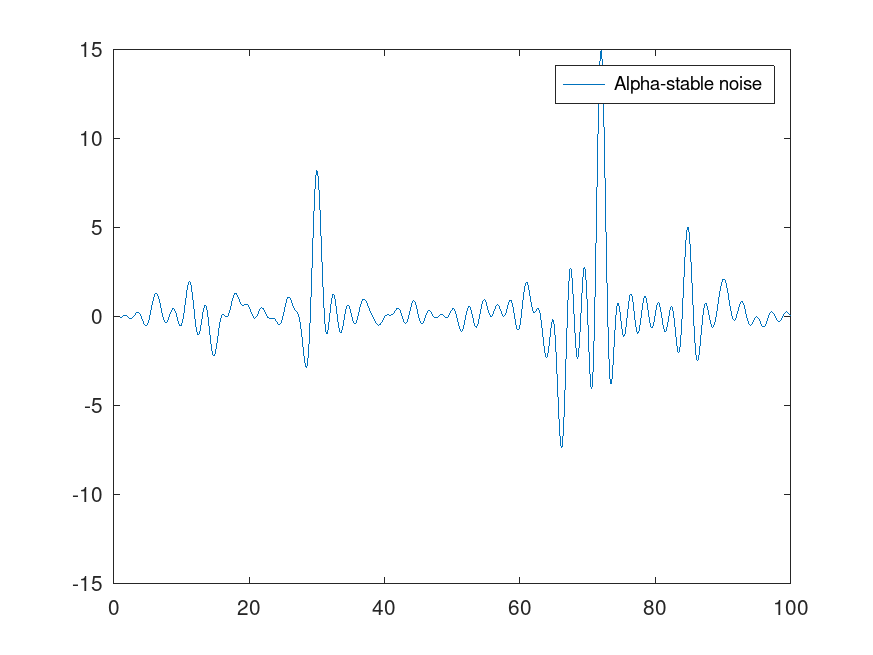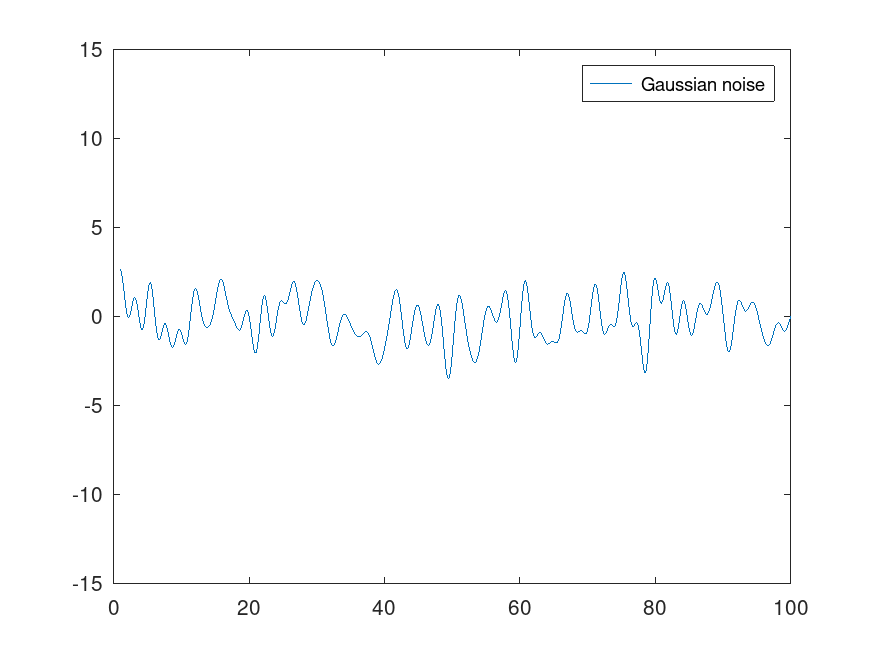## June – Gaussian vs. impulsive noise

Noise is often modeled as Gaussian. However, it can be an unrealistic model in certain scenarios if the “rare events” are “less rare” than in the Gaussian distribution. Particularly, this is the case with fat tailed distributions, that have a significant mass in the tail distributions. Other impulsive noise distributions (that are not necessarily fat-tailed or heavy-tailed) are the Middleton distributions, which are derived for the envelope of non-Gaussian electromagnetic noise.

Particularly, the-stable distributions are used as noise models in various applications including economics and electromagnetic communications. Also the normal distribution belongs to the family of-stable distribution when the stability parameter, being the only distribution in the family having a well-defined variance. With values, even the mean is ill-defined, and the qualitative difference to the normal distribution is clear: imagine investing money in a share that has an fixed expected profit value – say, 10 euros in a year – versus investing to a share that of which value fluctuates so rapidly that the expected profit (mean profit over time) can be – in a sense – anything.

In the following, we compare the Gaussian and-stable noises. That is, the in-phase and quadrature components are distributed as Gaussian distribution or-stable distribution with, accordingly.

The following GNU Octave code produces plots of bandwidth limited analog Gaussian and-stable noises. The function digitaltoanalog is given my May's blog entry. Also, remember to import to statistical package to Octave.

clear all;
close all;

%%Inverse of the alpha stable CDF with alpha = 1.
invalphaCDF = @(x) tan(0.5*(-1 + 2*x)*pi);
%%Generate N Alpha-stable samples.
N = 100;
alphasamples = invalphaCDF(unifrnd(0,1,1,N));

%%Generate normal samples.
normalsamples = normrnd(0,1,1,N);
%%Convert to analog signal.
T = 1 : 0.1 : N;
alphasamples = digitaltoanalog(T, alphasamples, 1);
normalsamples = digitaltoanalog(T, normalsamples, 1);

alphasamples = alphasamples/(mean(abs(alphasamples))); %Normalize by mean instantaneous amplitude.
normalsamples = normalsamples/(mean(abs(normalsamples)));
figure(1)
plot(T, alphasamples)
legend('Alpha-stable noise')
axis([0 100 -15 15])
figure(2)
plot(T, normalsamples)
legend('Gaussian noise')
axis([0 100 -15 15])Looking the pictures, it is easy to understand where the term “impulsive noise” comes from.

It is also interesting to compare the audiolized noise-signals: my Soundcloud.

References:

• Samorodnitsky, Gennady., and Murad S. Taqqu. Stable Non-Gaussian Random Processes : Stochastic Models with Infinite Variance. New York: Chapman & Hall, 1994. Print.
• Middleton, David. Statistical-Physical Models of Man-made and Natural Radio Noise parts I - , 1976 - .